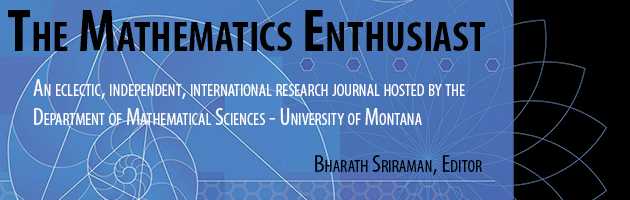•
•17

1

#### Abstract

In mathematical problem solving, students’ written work mostly reveals their mathematical algorithm skills and has very little information about their reasoning skills of the problem solving process. This study extends the features of mathematical writing that integrate the language and mathematical thinking to increase students’ mathematical problem solving skills. The main feature of this study is the use of mathematics writing workbook as a practical approach to guide the students in the problem solving process. Thirty Foundation students in Engineering participated in a six weeks of writing to solve mathematical problems. An exploratory case study analysis was used to examine the written contents of the participants’ mathematical writing workbook, the performance of their formal test as well as their perceptions of mathematical writing. The trace of work in the workbooks showed that mathematical writing has somehow given some impact on these students to visualize, aware and recognize their problem solving behaviors in words.

239

273

#### Digital Object Identifier (DOI)

10.54870/1551-3440.1486

COinS